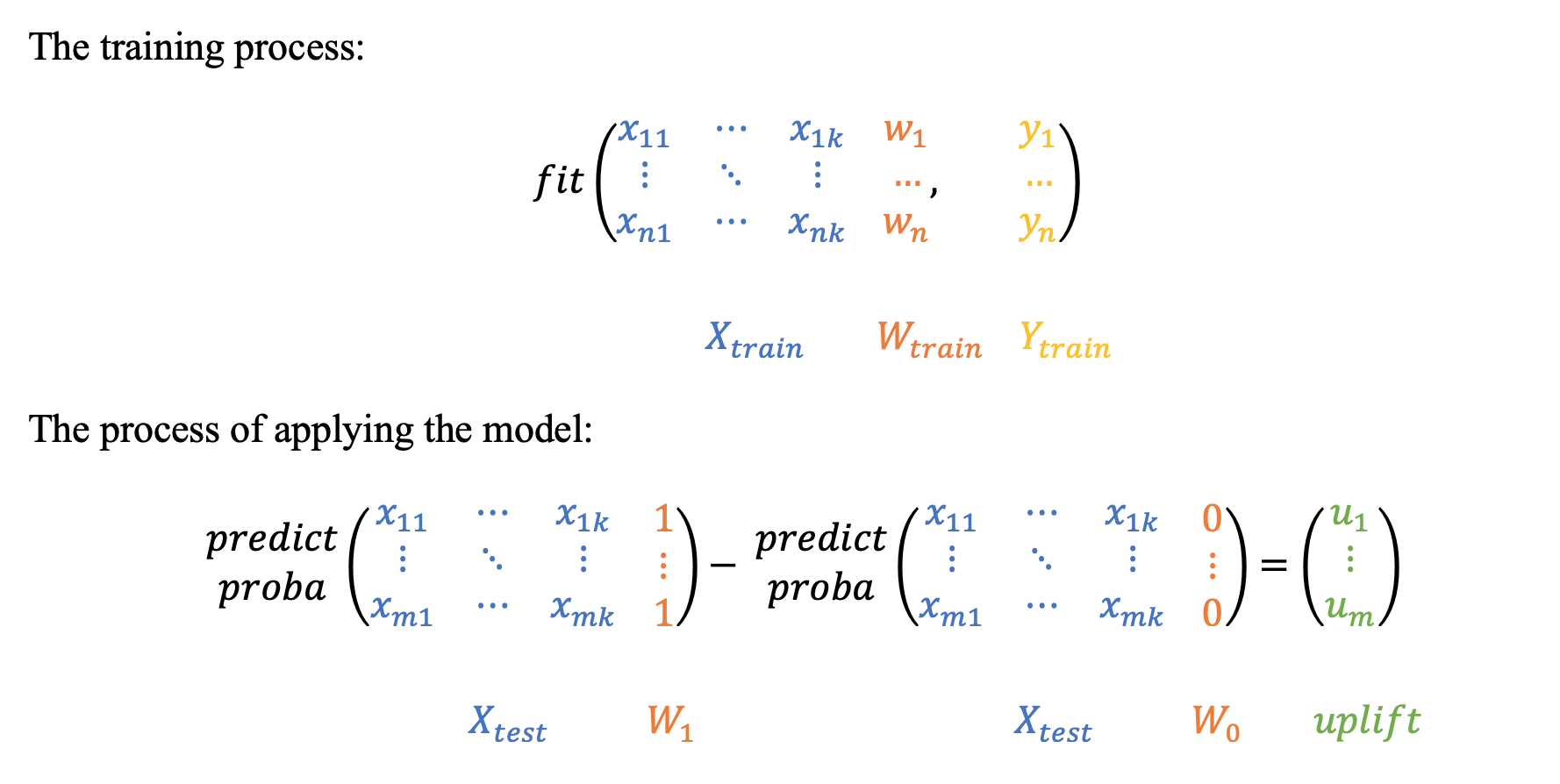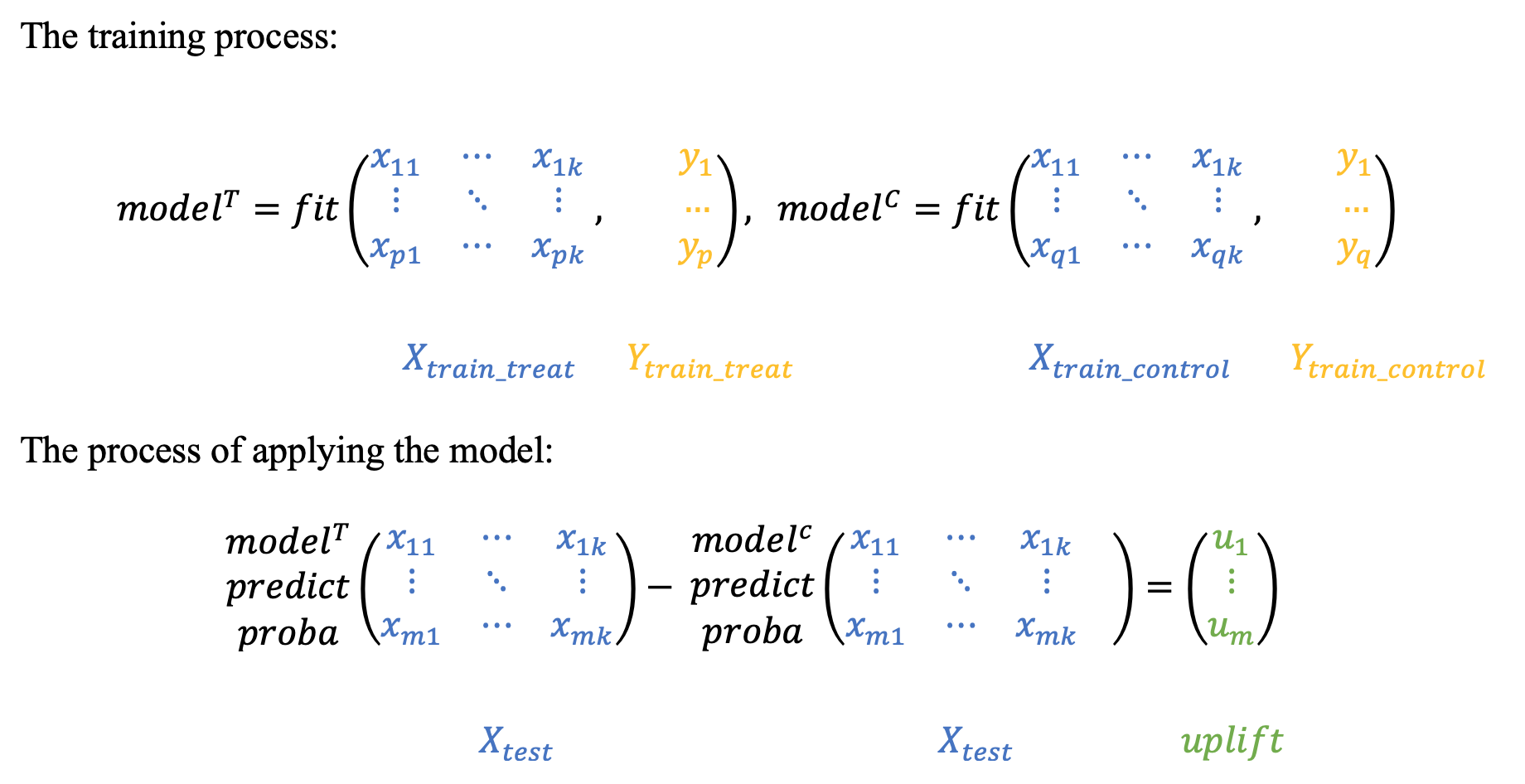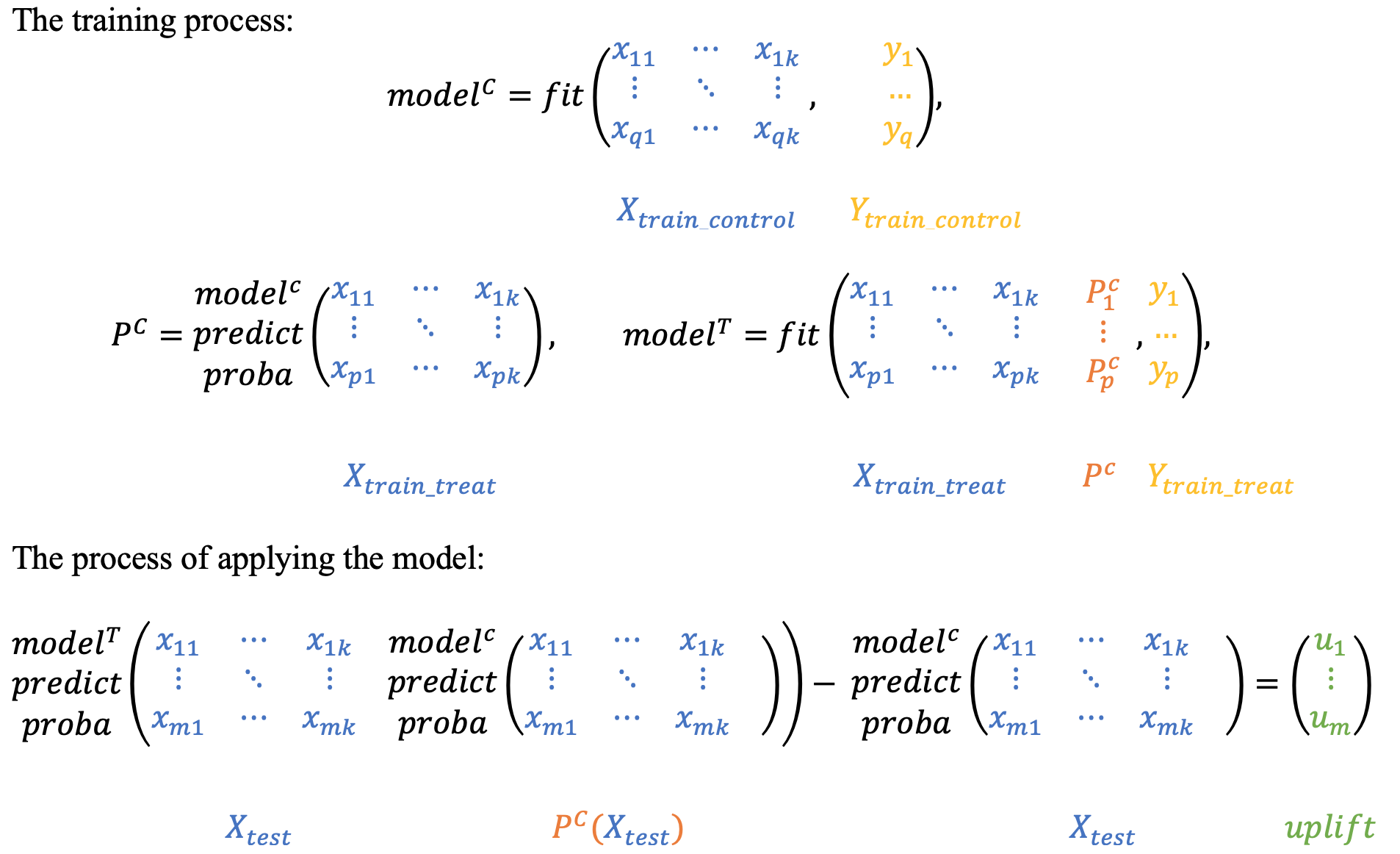# Models (sklift.models)¶

## 1. Approaches with the same model¶

### 1.1 One model with treatment as feature¶

The simplest and most intuitive solution: the model is trained on union of two groups, with the binary communication flag acting as an additional feature. Each object from the test sample is scored twice: with the communication flag equal to 1 and equal to 0. Subtracting the probabilities for each observation, we get the required uplift.class sklift.models.models.SoloModel(estimator)[source]

aka Treatment Dummy approach, or Single model approach, or S-Learner.

Fit solo model on whole dataset with ‘treatment’ as an additional feature.

For each test example calculate predictions on new set twice: with treatment == ‘1’ and with treatment == ‘0’. After that calculate uplift as a delta between these predictions.

Return delta of predictions for each example.

See more details about SoloModel in documentation.

Parameters: estimator (estimator object implementing 'fit') – The object to use to fit the data.
trmnt_preds_

Estimator predictions on samples when treatment.

Type: array-like, shape (n_samples, )
ctrl_preds_

Estimator predictions on samples when control.

Type: array-like, shape (n_samples, )

Example:

# import approach
from sklift.models import SoloModel
# import any estimator adheres to scikit-learn conventions
from catboost import CatBoostClassifier

sm = SoloModel(CatBoostClassifier(verbose=100, random_state=777))  # define approach
sm = sm.fit(X_train, y_train, treat_train, estimator_fit_params={{'plot': True})  # fit the model
uplift_sm = sm.predict(X_val)  # predict uplift


References

Lo, Victor. (2002). The True Lift Model - A Novel Data Mining Approach to Response Modeling in Database Marketing. SIGKDD Explorations. 4. 78-86.

fit(X, y, treatment, estimator_fit_params=None)[source]

Fit the model according to the given training data.

For each test example calculate predictions on new set twice: by the first and second models. After that calculate uplift as a delta between these predictions.

Return delta of predictions for each example.

Parameters: X (array-like, shape (n_samples, n_features)) – Training vector, where n_samples is the number of samples and n_features is the number of features. y (array-like, shape (n_samples,)) – Target vector relative to X. treatment (array-like, shape (n_samples,)) – Binary treatment vector relative to X. estimator_fit_params (dict, optional) – Parameters to pass to the fit method of the estimator. self object
predict(X)[source]

Perform uplift on samples in X.

Parameters: X (array-like, shape (n_samples, n_features)) – Training vector, where n_samples is the number of samples and n_features is the number of features. uplift array (shape (n_samples,))

### 1.2 Class Transformation¶

Warning

This approach is only suitable for classification problem

Quite an interesting and mathematically confirmed approach to the construction of the model, presented back in 2012. The method is to predict a slightly changed target:

$z_i = y_i * w_i + (1 - y_i) * (1 - w_i), где$
• $$z_i$$ - new target for $$i$$ customer;
• $$y_i$$ - old target $$i$$ customer;
• $$w_i$$ - treatment flag $$i$$ customer.

In other words, the new class is 1 if we know that on a particular observation, the result in the interaction would be as good as in the control group if we could know the result in both groups:

$\begin{split}z_i = \begin{cases} 1, & \mbox{if } w_i = 1 \mbox{ and } y_i = 1 \\ 1, & \mbox{if } w_i = 0 \mbox{ and } y_i = 0 \\ 0, & \mbox{otherwise} \end{cases}\end{split}$

Let’s describe in more detail what is the probability of a new target variable:

$\begin{split}P(Z=1|X_1, ..., X_m) = \\ = P(Z=1|X_1, ..., X_m, W = 1) * P(W = 1|X_1, ..., X_m, ) + \\ + P(Z=1|X_1, ..., X_m, W = 0) * P(W = 0|X_1, ..., X_m, ) = \\ = P(Y=1|X_1, ..., X_m, W = 1) * P(W = 1|X_1, ..., X_m, ) + \\ + P(Y=0|X_1, ..., X_m, W = 0) * P(W = 0|X_1, ..., X_m, ).\end{split}$

We assume that $$W$$ does not depend on the attributes of $$X_1, ..., X_m$$, because otherwise the experiment design is not very well designed. Taking this, we have: $$P(W | X_1, ..., X_m, ) = P(W)$$ and

$\begin{split}P(Z=1|X_1, ..., X_m) = \\ = P^T(Y=1|X_1, ..., X_m) * P(W = 1) + \\ + P^C(Y=0|X_1, ..., X_m) * P(W = 0).\end{split}$

Also assume that $$P(W = 1) = P(W = 0) = \frac{1}{2}$$, i.e. during the experiment, the control and treatment groups were divided in equal proportions. Then we get the following:

$\begin{split}P(Z=1|X_1, ..., X_m) = \\ = P^T(Y=1|X_1, ..., X_m) * \frac{1}{2} + P^C(Y=0|X_1, ..., X_m) *\frac{1}{2} \Rightarrow \\ \Rightarrow 2 * P(Z=1|X_1, ..., X_m) = \\ = P^T(Y=1|X_1, ..., X_m) + P^C(Y=0|X_1, ..., X_m) = \\ = P^T(Y=1|X_1, ..., X_m) + 1 - P^C(Y=1|X_1, ..., X_m) \Rightarrow \\ \Rightarrow P^T(Y=1|X_1, ..., X_m) - P^C(Y=1|X_1, ..., X_m) = \\ = UPLIFT = 2 * P(Z=1|X_1, ..., X_m) - 1\end{split}$

Thus, by doubling the forecast of the new target and subtracting one from it, we get the value of the uplift itself, i.e.

$UPLIFT = 2 * P(Z=1) - 1$

Based on the assumption described above: $$P(W = 1) = P(W = 0) = \frac{1}{2}$$, this approach should be used only in cases where the number of clients with whom we have communicated is equal to the number of clients with whom there was no communication.

class sklift.models.models.ClassTransformation(estimator)[source]

aka Class Variable Transformation or Revert Label approach.

Redefine target variable, which indicates that treatment make some impact on target or did target is negative without treatment.

Z = Y * W + (1 - Y)(1 - W),

where Y - target, W - communication flag.

Then, Uplift ~ 2 * (Z == 1) - 1

Returns only uplift predictions.

See more details about ClassTransformation in documentation.

Parameters: estimator (estimator object implementing 'fit') – The object to use to fit the data.

Example:

# import approach
from sklift.models import ClassTransformation
# import any estimator adheres to scikit-learn conventions
from catboost import CatBoostClassifier

ct = ClassTransformation(CatBoostClassifier(verbose=100, random_state=777))  # define approach
ct = ct.fit(X_train, y_train, treat_train, estimator_fit_params={{'plot': True})  # fit the model
uplift_ct = ct.predict(X_val)  # predict uplift


References

Maciej Jaskowski and Szymon Jaroszewicz. Uplift modeling for clinical trial data. ICML Workshop on Clinical Data Analysis, 2012.

fit(X, y, treatment, estimator_fit_params=None)[source]

Fit the model according to the given training data.

Parameters: X (array-like, shape (n_samples, n_features)) – Training vector, where n_samples is the number of samples and n_features is the number of features. y (array-like, shape (n_samples,)) – Target vector relative to X. treatment (array-like, shape (n_samples,)) – Binary treatment vector relative to X. estimator_fit_params (dict, optional) – Parameters to pass to the fit method of the estimator. self object
predict(X)[source]

Perform uplift on samples in X.

Parameters: X (array-like, shape (n_samples, n_features)) – Training vector, where n_samples is the number of samples and n_features is the number of features. uplift array (shape (n_samples,))

## 2. Approaches with two models¶

The two-model approach can be found in almost any uplift modeling work, and is often used as a baseline. However, the use of two models can lead to some unpleasant consequences: if the training will be used fundamentally different models or the nature of the data of the test and control groups will be very different, then the returned models will not be comparable with each other. As a result, the calculation of the uplift will not be completely correct. To avoid this effect, it is necessary to calibrate the models so that their scores can be interpolated as probabilities. Calibration of model probabilities is well described in the scikit-learn documentation.

### 2.1 Two independent models¶

Hint

In sklift this approach corresponds to the TwoModels class and the vanilla method.

As the name implies, the approach is to model the conditional probabilities of the treatment and control groups separately. The articles argue that this approach is rather weak, since both models focus on predicting the result separately and can therefore skip the “weaker” differences in the samples.### 2.2 Two dependent models¶

The dependent data representation approach is based on the classifier chain method originally developed for multi-class classification problems. The idea is that if there are $$L$$ different labels, you can build $$L$$ different classifiers, each of which solves the problem of binary classification and in the learning process, each subsequent classifier uses the predictions of the previous ones as additional features. The authors of this method proposed to use the same idea to solve the problem of uplift modeling in two stages.

Hint

In sklift this approach corresponds to the TwoModels class and the ddr_control method.

At the beginning we train the classifier based on control data:

$P^C = P(Y=1| X, W = 0),$

then we will perform the $$P_C$$ predictions as a new feature for training the second classifier on test data, thus effectively introducing a dependency between the two data sets:

$P^T = P(Y=1| X, P_C(X), W = 1)$

To get the uplift for each observation, calculate the difference:

$uplift(x_i) = P^T (x_i, P_C(x_i)) - P^C(x_i)$

Intuitively, the second classifier studies the difference between the expected result in the test and the control, i.e. the uplift itself.Similarly, you can first train the $$P_T$$ classifier and then use its predictions as a trait for the $$P_C$$ classifier.

Hint

In sklift this approach corresponds to the TwoModels class and the ddr_treatment method.

class sklift.models.models.TwoModels(estimator_trmnt, estimator_ctrl, method='vanilla')[source]

aka naïve approach, or difference score method, or double classifier approach. Fit two separate models: on the treatment data and on the control data.

See more details about TwoModels in documentation.

Parameters: estimator_trmnt (estimator object implementing 'fit') – The object to use to fit the treatment data. estimator_ctrl (estimator object implementing 'fit') – The object to use to fit the control data. method (string, ‘vanilla’, ’ddr_control’ or ‘ddr_treatment’, default='vanilla') – Specifies the approach: * ‘vanilla’ - two independent models * ’ddr_control’ - dependent data representation (First train control estimator) * ’ddr_treatment’ - dependent data representation (First train treatment estimator)
trmnt_preds_

Estimator predictions on samples when treatment.

Type: array-like, shape (n_samples, )
ctrl_preds_

Estimator predictions on samples when control.

Type: array-like, shape (n_samples, )

Example:

# import approach
from sklift.models import TwoModels
# import any estimator adheres to scikit-learn conventions
from catboost import CatBoostClassifier

estimator_trmnt = CatBoostClassifier(silent=True, thread_count=2, random_state=42)
estimator_ctrl = CatBoostClassifier(silent=True, thread_count=2, random_state=42)

# define approach
tm_ctrl = TwoModels(
estimator_trmnt=estimator_trmnt,
estimator_ctrl=estimator_ctrl,
method='ddr_control'
)

# fit the models
tm_ctrl = tm_ctrl.fit(
X_train, y_train, treat_train,
estimator_trmnt_fit_params={'cat_features': cat_features},
estimator_ctrl_fit_params={'cat_features': cat_features}
)
uplift_tm_ctrl = tm_ctrl.predict(X_val)  # predict uplift

References

Betlei, Artem & Diemert, Eustache & Amini, Massih-Reza. (2018). Uplift Prediction with Dependent Feature Representation in Imbalanced Treatment and Control Conditions: 25th International Conference, ICONIP 2018, Siem Reap, Cambodia, December 13–16, 2018, Proceedings, Part V. 10.1007/978-3-030-04221-9_5.

Zhao, Yan & Fang, Xiao & Simchi-Levi, David. (2017). Uplift Modeling with Multiple Treatments and General Response Types. 10.1137/1.9781611974973.66.

fit(X, y, treatment, estimator_trmnt_fit_params=None, estimator_ctrl_fit_params=None)[source]

Fit the model according to the given training data.

For each test example calculate predictions on new set twice: by the first and second models. After that calculate uplift as a delta between these predictions.

Return delta of predictions for each example.

Parameters: X (array-like, shape (n_samples, n_features)) – Training vector, where n_samples is the number of samples and n_features is the number of features. y (array-like, shape (n_samples,)) – Target vector relative to X. treatment (array-like, shape (n_samples,)) – Binary treatment vector relative to X. estimator_trmnt_fit_params (dict, optional) – Parameters to pass to the fit method of the treatment estimator. estimator_ctrl_fit_params (dict, optional) – Parameters to pass to the fit method of the control estimator. self object
predict(X)[source]

Perform uplift on samples in X.

Parameters: X (array-like, shape (n_samples, n_features)) – Training vector, where n_samples is the number of samples and n_features is the number of features. uplift array (shape (n_samples,))